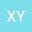loading page

A new type of S-N equation and its application to multiaxial fatigue life prediction
•## Abstract

The S-N equation is one of the most important equations in fatigue model investigation. A majority of fatigue models, including multiaxial fatigue model and mean effect models, are established on the basis of the S-N equation. Obviously, an accuracy of the S-N equation is very important. Taking into account that the S-N equation is, in fact, an empirical one in which the material constants are determined by numerical fitting fatigue experimental data, in this paper, the S-N equation can be improved, by further processing these fatigue experimental data, to present a new type of S-N equation that is more accurate than the S-N equation. The new type of S-N equation is called a similar S-N equation in this paper. By using a large number of experimental data of metallic materials reported in literature, an accuracy of the similar S-N equation has been proven.